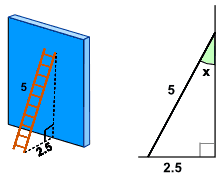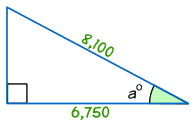# Finding an Angle in a Right Angled Triangle

## Angle from Any Two Sides

We can find an unknown angle in a right-angled triangle, as long as we know the lengths of two of its sides.### Example

The ladder leans against a wall as shown.

What is the angle between the ladder and the wall?

The answer is to use Sine, Cosine or Tangent!

But which one to use? We have a special phrase "SOHCAHTOA" to help us, and we use it like this:

Step 1: find the names of the two sides we know• Opposite is opposite the angle,
• and the longest side is the Hypotenuse.

### Example: in our ladder example we know the length of:

• the side Opposite the angle "x", which is 2.5
• the longest side, called the Hypotenuse, which is 5

Step 2: now use the first letters of those two sides (Opposite and Hypotenuse) and the phrase "SOHCAHTOA" to find which one of Sine, Cosine or Tangent to use:

 SOH... Sine: sin(θ) = Opposite / Hypotenuse ...CAH... Cosine: cos(θ) = Adjacent / Hypotenuse ...TOA Tangent: tan(θ) = Opposite / Adjacent

In our example that is Opposite and Hypotenuse, and that gives us “SOHcahtoa”, which tells us we need to use Sine.

Step 3: Put our values into the Sine equation:

Sin (x) = Opposite / Hypotenuse = 2.5 / 5 = 0.5

Step 4: Now solve that equation!

sin(x) = 0.5

Next (trust me for the moment) we can re-arrange that into this:

x = sin-1(0.5)

And then get our calculator, key in 0.5 and use the sin-1 button to get the answer:

x = 30°

### But what is the meaning of sin-1 … ?

Well, the Sine function "sin" takes an angle and gives us the ratio "opposite/hypotenuse",But sin-1 (called "inverse sine") goes the other way ...
... it takes the ratio "opposite/hypotenuse" and gives us an angle.

### Example:

• Sine Function: sin(30°) = 0.5
• Inverse Sine Function: sin-1(0.5) = 30°On the calculator press one of the following (depending on your brand of calculator): either '2ndF sin' or 'shift sin'.

On your calculator, try using sin and sin-1 to see what results you get!

Also try cos and cos-1. And tan and tan-1.
Go on, have a try now.

## Step By Step

These are the four steps we need to follow:

• Step 1 Find which two sides we know – out of Opposite, Adjacent and Hypotenuse.
• Step 2 Use SOHCAHTOA to decide which one of Sine, Cosine or Tangent to use in this question.
• Step 3 For Sine calculate Opposite/Hypotenuse, for Cosine calculate Adjacent/Hypotenuse or for Tangent calculate Opposite/Adjacent.
• Step 4 Find the angle from your calculator, using one of sin-1, cos-1 or tan-1

## Examples

Let’s look at a couple more examples:### Example

Find the angle of elevation of the plane from point A on the ground.

• Step 1 The two sides we know are Opposite (300) and Adjacent (400).
• Step 2 SOHCAHTOA tells us we must use Tangent.
• Step 3 Calculate Opposite/Adjacent = 300/400 = 0.75
• Step 4 Find the angle from your calculator using tan-1

Tan x° = opposite/adjacent = 300/400 = 0.75

tan-1 of 0.75 = 36.9° (correct to 1 decimal place)

Unless you’re told otherwise, angles are usually rounded to one place of decimals.### Example

Find the size of angle a°

• Step 1 The two sides we know are Adjacent (6,750) and Hypotenuse (8,100).
• Step 2 SOHCAHTOA tells us we must use Cosine.
• Step 3 Calculate Adjacent / Hypotenuse = 6,750/8,100 = 0.8333
• Step 4 Find the angle from your calculator using cos-1 of 0.8333:

cos a° = 6,750/8,100 = 0.8333

cos-1 of 0.8333 = 33.6° (to 1 decimal place)

250, 1500, 1501, 1502, 251, 1503, 2349, 2350, 2351, 3934